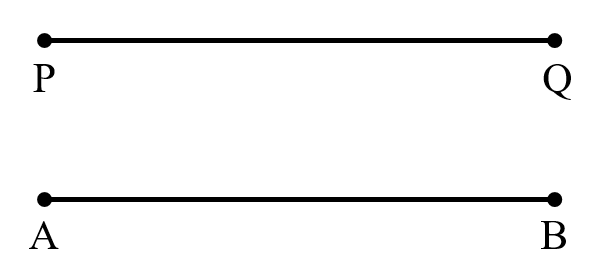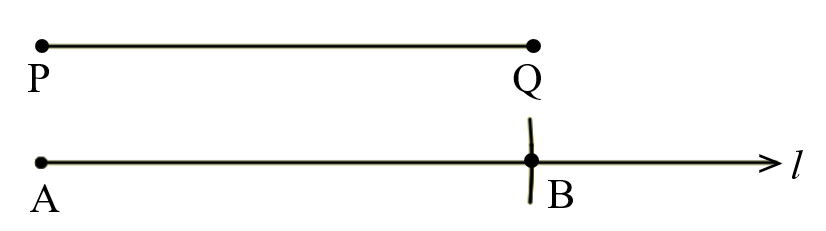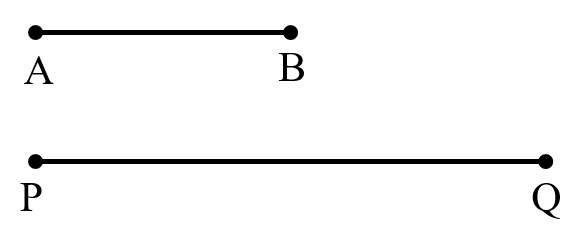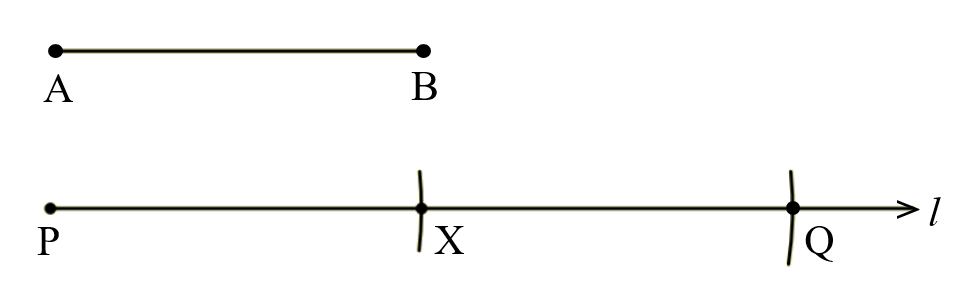# vs.eyeandcontacts.com

## Chapter 14 Practical Geometry Exercise 14.3

Question 1: Draw any line segment PQ. Without measuring line segment PQ, construct a copy of line segment PQ.

Given
line segment PQ

Rough figureActual figureSteps of Construction
1. Draw line segment PQ whose length is unknown.
2. Measure the line segment PQ with a compass.
3. Draw a line l. Mark point A on the line l.
4. With A as centre, draw an arc to cut line l at B.
5. Therefore, AB is the required line segment.

Question 2: Given some line segment AB, whose length you do not know, construct line segment PQ such that the length of line segment PQ is twice that of line segment AB.

Given
line segment AB

Rough figureActual figureSteps of Construction
1. Draw line segment AB whose length is unknown.
2. Measure the line segment AB with a compass.
3. Draw a line l. Mark point P on the line l.
4. With P as centre, draw an arc to cut the line l at X.
5. With X as centre, draw another arc to cut the line l at Q.
6. Therefore, PQ is the required line segment.